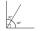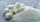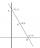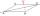# Grade - math word problems

1. Flour2 kg of flour costs 100 CZK. How much does it cost half a kilogram?
2. Electric energyThere are 875 identical light bulbs in the sports hall lighting for 2 hours. How long does the same amount of electricity consume 100 such bulbs?
3. CommissionDaniel works at a nearby electronics store. He makes a commission of 15%, percent on everything he sells. If he sells a laptop for 293.00\$ how much money does Daniel make in commission?
4. Complementary angles 2Two complementary angles are (x+4) and (2x - 7) find the value of x
5. Test pointsIf you earned 80% of the possible 40 points, how many points did you miss to get 100%?
6. Combinations 66 purses 9 flaps 12 straps Every combination must include 1 purse, 1 flap, and 1 strap. How many are possible combinations?
7. Ruler and compassUse a ruler and compass to construct a triangle ABC with AB 5cm BAC 60° and ACB 45°.
8. Find twoFind two consecutive natural numbers whose product is 1 larger than their sum. Searched numbers expressed by a fraction whose numerator is the difference between these numbers and the denominator is their sum.
9. Equilateral triangleA square is inscribed into an equilateral triangle with a side of 10 cm. Calculate the length of the square side.
10. Fruit juiceFruit juice contains 37.5% sugar. How many percents of sugar will be in the flavored mineral that we prepare from 100 grams of fruit juice and 1.4 liters of mineral? (1 liter = 1 kg)
11. Two numbers 6Fill two natural numbers a, b: 7 + blank- blank = 5
12. Coordinate axesDetermine the area of the triangle given by line -7x+7y+63=0 and coordinate axes x and y.
13. Cube edgesIf the edge length of the cube increases by 50%, how does the volume of this cube increase?
14. Tallest and shortestJenn is the tallest on the team. He is 1 1/2 times as tall as the shortest girl whom is 4 1/4 feet tall. How tall is Jebb?
15. Right triangular prismWe have cuboid with a base and dimensions of 12 cm and 5 cm and height of 4 cm. The tablecloth cut it into two identical triangular prisms with right triangular bases. The surface of the created prisms was painted with color. Calculate the surface area of
16. Bitoo and ReenaBitoo ate 3/5 part of an apple and the remaining part was eaten by his sister Reena. How much part of an apple did Renna eat? Who had the larger share? By how much?
17. The priceThe price of the land increased by 17%. What was the original price of the land if it now costs 46800 €?
18. ConstructConstruct a rhombus ABCD, if the size of the diagonal AC is 6 cm and diagonal BD 8 cm long.
19. ZdeněkZdeněk picked up 15 l of water from a 100-liter full-water barrel. Write a fraction of what part of Zdeněk's water he picked.
20. Father and sonWhen I was 11, my father was 35 years old. Today, my father has three times more years than me. How old is she?

Do you have an interesting mathematical word problem that you can't solve it? Enter it, and we can try to solve it.

To this e-mail address, we will reply solution; solved examples are also published here. Please enter the e-mail correctly and check whether you don't have a full mailbox.

Please do not submit problems from current active competitions such as Mathematical Olympiad, correspondence seminars etc...